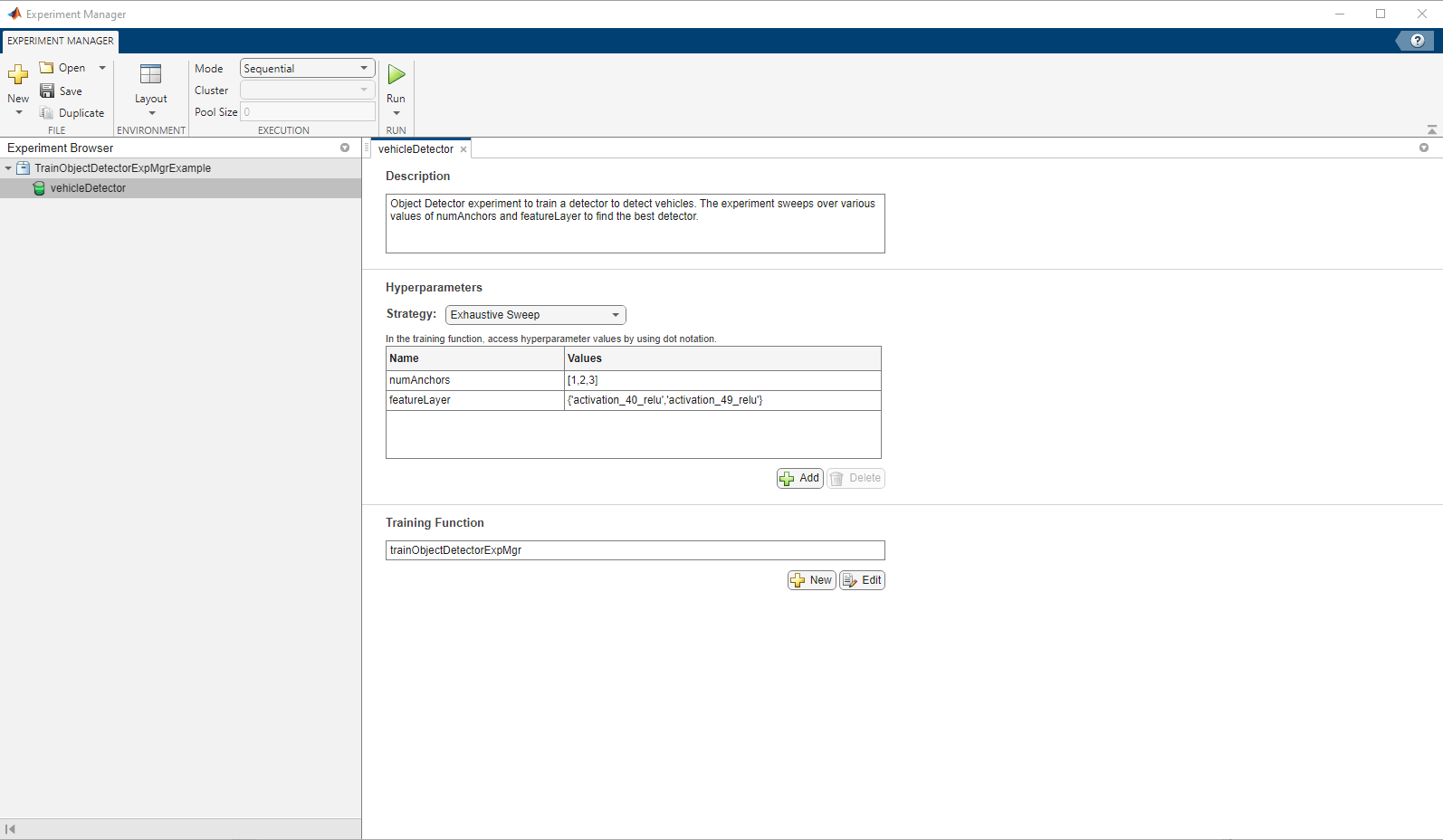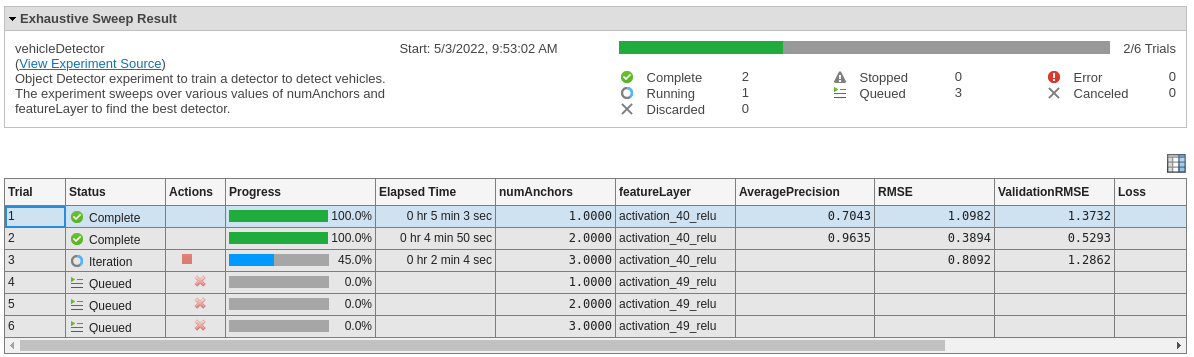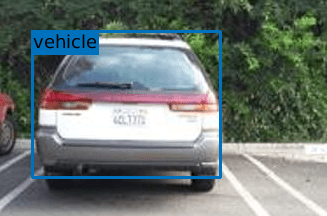# Train Object Detectors in Experiment Manager

This example shows how to use Experiment Manager app to find optimal training options for object detectors by sweeping through a range of hyperparameter values.

### Overview

The Experiment Manager app enables you to create deep learning experiments to train object detectors under multiple initial conditions and compare the results. In this example, you will use the Experiment Manager app to train a YOLO v2 object detector to detect vehicles from traffic images. You will sweep over the number-of-anchors and different choices of feature extraction layer to obtain the best performing object detector. Experiment Manager trains the object detector using every combination of hyperparameter values specified in the hyperparameter table. Note that in this experiment you will run trials over different values of `numAnchors` and `featureLayer` for simplicity. To find the optimum object detector, specify experiment trials to sweep across additional hyperparameters such as learning rate, mini-batch size, and image size.

### Open Experiment

First, open the example. Experiment Manager loads the project with a custom object detector experiment that you can inspect and run.The Hyperparameter section allows you to set the hyperparameters you wish to sweep over.

The Training Function section allows you to specify a custom training script to use for the experiment. This example uses `vehicleDetectorExperiment` function which loads in the data, model, and performs training. Details of the script are described in this section. The complete function is listed under the Supporting Functions section.

Load in the data using the utility function `splitVehicleData` . This example uses a small vehicle dataset that contains 295 images. Many of these images come from the Caltech Cars 1999 and 2001 data sets, available at the Caltech Computational Vision website, created by Pietro Perona, and used with permission. Each image contains one or two labeled instances of a vehicle.

```output.trainedNet = []; output.ap = []; output.executionEnvironment = "auto"; monitor.Info = "AveragePrecision"; [trainingData,validationData,testData] = splitVehicleData;```

Apply augmentations and preprocessing on the training dataset. For the validation and test dataset only preprocessing is needed.

```inputSize = [224 224]; augmentedTrainingData = transform(trainingData, @augmentData); preprocessedTrainingData = transform(augmentedTrainingData, @(data)preprocessData(data, inputSize)); preprocessedValidationData = transform(validationData, @(data)preprocessData(data, inputSize)); preprocessedTestData = transform(testData, @(data)preprocessData(data, inputSize));```

For this example, you will sweep over the number of anchor boxes for training. Anchor Boxes for Object Detection are defined to capture the scale and aspect ratio of specific object classes you want to detect and are typically chosen based on object sizes in your training datasets. Multiple anchor boxes enable the object detector to detect objects of different sizes. The shape, scale, and number of anchor boxes impact the efficiency and accuracy of the detectors. A large number of anchor boxes decrease the runtime performance of the detector. The `estimateAnchorBoxes` function uses the hyperparameter (`params.numAnchors)` passed by the Experiment Manager during each trial. This changes the number of anchor boxes estimated during each trial. For more information about the anchor box estimation see, Estimate Anchor Boxes From Training Data.

`aboxes = estimateAnchorBoxes(preprocessedTrainingData, params.numAnchors);`

Set the training options.

```opts = trainingOptions("rmsprop", ... InitialLearnRate=0.001, ... MiniBatchSize=16, ... MaxEpochs=20, ... LearnRateSchedule="piecewise", ... LearnRateDropPeriod=5, ... VerboseFrequency=30, ... L2Regularization=0.001, ... ValidationData=preprocessedValidationData, ... ValidationFrequency=50);```

Create a YOLOv2 object detector using `yolov2Layers` with Resnet50 backbone. For this example, you will also sweep over different feature extraction layers. Different feature extraction layers correspond to different amounts of downsampling. There is a good trade-off between spatial resolution and the strength of the extracted features, as features extracted further down the network encode stronger image features at the cost of spatial resolution. Set `featureLayer` to `params.featureLayer` to accept the input from Experiment Manager. You can visualize the network to identify different feature layers using `analyzeNetwork` or D|eepNetworkDesigner| from Deep Learning Toolbox&trade.

```numClasses = 1; inputSize = [224 224 3]; network = resnet50(); featureLayer = params.featureLayer; lgraph = yolov2Layers(inputSize, numClasses, aboxes, network, featureLayer);```

The network will be trained using the `trainYOLOv2ObjectDetector` function with the ExperimentMonitor name-value pair set to `monitor`. Setting this name value-pair allows the trainer to feed the training statistics back to Experiment Monitor at regular intervals.

```detector = trainYOLOv2ObjectDetector(preprocessedTrainingData, lgraph,... opts, ExperimentMonitor=monitor);```

Assess the trained object detector on the validation set by computing the average precision score. Precision is a ratio of true positive instances to all positive instances of objects in the detector, based on the ground truth.

```results = detect(detector,preprocessedTestData, MiniBatchSize=4); [ap, ~, ~] = evaluateDetectionPrecision(results, preprocessedTestData);```

Update the average precision metric in Experiment Manager and package the trained detector and average precision score into the output struct.

```updateInfo(monitor, AveragePrecision=ap); output.trainedNet = detector; output.ap = ap;```

### Run Experiment

Click the Run button on the Experiment Manager toolstrip to start the training trials.

When you run the experiment, Experiment Manager trains the network defined by the training function six times. Each trial uses a unique combination of `numAnchors` and `featureLayer` specified in the hyperparameter table. By default, Experiment Manager runs one trial at a time. If you have Parallel Computing Toolbox&trade, you can run multiple trials at the same time. For best results, before you run your experiment, start a parallel pool with as many workers as GPUs.

A table of results displays the training loss for each trial.### Export results

Export the best-trained detector to the workspace:

• Select the trial with the lowest loss score.

• On the Experiment Manager toolstrip, click Export.

• In the dialog window, enter the name of a workspace variable for the exported training output. The default name is trainingOutput.

Visualize the results of the trained detector by calling the `runDetectorOnTestImage` function.

`runDetectorOnTestImage(trainingOutput)`### Appendix 1: Training Function

The `vehicleDetectorExperiment` function specifies the training data, network architecture, training options, and training procedure used by the experiment.

This function takes in two arguments,

• `params` is a structure with fields from the Experiment Manager hyperparameter table.

• `monitor` is an `experiments.Monitor` (Deep Learning Toolbox) object that you can use to track the progress of the training, update information fields in the results table, record values of the metrics used by the training, and produce training plots.

The output of this function is a struct that contains the trained detector network, the execution environment, and Average Precision metrics for the trained network. Experiment Manager saves this output, so you can export it to the MATLAB workspace when the training is complete.

```function output = trainObjectDetectorExpMgr(params,monitor) output.trainedNet = []; output.ap = []; output.executionEnvironment = "auto"; % Add AveragePrecision field to the Experiment Manager. monitor.Info = "AveragePrecision"; % Load data and split it into training, validation and test sets [trainingData,validationData,testData] = splitVehicleData; % Augment and preprocess the data inputSize = [224 224]; augmentedTrainingData = transform(trainingData,@augmentData); preprocessedTrainingData = transform(augmentedTrainingData, @(data)preprocessData(data, inputSize)); preprocessedValidationData = transform(validationData, @(data)preprocessData(data, inputSize)); preprocessedTestData = transform(testData, @(data)preprocessData(data, inputSize)); % Setup the training options opts = trainingOptions("rmsprop",... InitialLearnRate=0.001,... MiniBatchSize=16,... MaxEpochs=20,... LearnRateSchedule="piecewise",... LearnRateDropPeriod=5,... VerboseFrequency=30, ... L2Regularization=0.001,... ValidationData=preprocessedValidationData,... ValidationFrequency=50); % Construct the YOLO v2 detector numClasses = 1; inputSize = [224 224 3]; network = resnet50(); featureLayer = params.featureLayer; % Estimate anchor boxes by using numAnchors parameter from the Experiment Manager aboxes = estimateAnchorBoxes(preprocessedTrainingData, params.numAnchors); lgraph = yolov2Layers(inputSize,numClasses,aboxes,network, featureLayer); % Train YOLOv2 detector [detector, info] = trainYOLOv2ObjectDetector(preprocessedTrainingData, lgraph, opts, ExperimentMonitor=monitor); % Capture Average Precision result with the output results = detect(detector,preprocessedTestData, MiniBatchSize=4); [ap, ~, ~] = evaluateDetectionPrecision(results, preprocessedTestData); updateInfo(monitor, AveragePrecision=ap); output.trainedNet = detector; output.ap = ap; output.info = info; end ```

### Appendix 2: Data Preprocessing Functions

`augmentData` function

The `augmentData` function returns augmented images for training.

```function B = augmentData(A) % Apply random horizontal flipping, and random X/Y scaling. Boxes that get % scaled outside the bounds are clipped if the overlap is above 0.25. Also, % jitter image color. B = cell(size(A)); I = A{1}; sz = size(I); if numel(sz)==3 && sz(3) == 3 I = jitterColorHSV(I,... Contrast=0.2,... Hue=0,... Saturation=0.1,... Brightness=0.2); end % Randomly flip and scale image. tform = randomAffine2d(XReflection=true, Scale=[1 1.1]); rout = affineOutputView(sz, tform, BoundsStyle="CenterOutput"); B{1} = imwarp(I, tform, OutputView=rout); % Sanitize box data, if needed. A{2} = helperSanitizeBoxes(A{2}, sz); % Apply same transform to boxes. [B{2},indices] = bboxwarp(A{2}, tform, rout, OverlapThreshold=0.25); B{3} = A{3}(indices); % Return original data only when all boxes are removed by warping. if isempty(indices) B = A; end end ```

preprocessData function

The `preprocessData` function rescales the images and the bounding boxes according to the target size.

```function data = preprocessData(data, targetSize) % Resize image and bounding boxes to the targetSize. sz = size(data{1}, [1 2]); scale = targetSize(1:2)./sz; data{1} = imresize(data{1}, targetSize(1:2)); % Sanitize box data, if needed. data{2} = helperSanitizeBoxes(data{2}, sz); % Resize boxes to new image size. data{2} = bboxresize(data{2}, scale); end ```
```% helperSanitizeBoxes Sanitize box data. % This example helper is used to clean up invalid bounding box data. Boxes % with values <= 0 are removed. % % If none of the boxes are valid, this function passes the data through to % enable downstream processing to issue proper errors. function boxes = helperSanitizeBoxes(boxes, ~) persistent hasInvalidBoxes valid = all(boxes > 0, 2); if any(valid) if ~all(valid) && isempty(hasInvalidBoxes) % Issue one-time warning about removing invalid boxes. hasInvalidBoxes = true; warning('Removing ground truth bouding box data with values <= 0.') end boxes = boxes(valid,:); end end ```

The `splitVehicleData` function loads the data and splits it into training, validation, and test datastores.
```function [dsTrain,dsVal,dsTest] = splitVehicleData() outputDir = fullfile(tempdir,'vehicleImages'); if ~exist(outputDir,'dir') % Unzip images and load the lables unzip('vehicleDatasetImages.zip', fullfile(tempdir)); end data = load('vehicleDatasetGroundTruth.mat'); vehicleDataset = data.vehicleDataset; % Load the list of image files vehicleDataset.imageFilename = fullfile(tempdir,vehicleDataset.imageFilename); rng(0); shuffledIndices = randperm(height(vehicleDataset)); idx = floor(0.6 * length(shuffledIndices) ); % Create a training, validation and test indices trainingIdx = 1:idx; validationIdx = idx+1 : idx + 1 + floor(0.1 * length(shuffledIndices) ); testIdx = validationIdx(end)+1 : length(shuffledIndices); % Load data using imageDatastore and boxLabelDatastore imds = imageDatastore(vehicleDataset{:,'imageFilename'}); blds = boxLabelDatastore(vehicleDataset(:,'vehicle')); allData = combine(imds,blds); dsTrain = subset(allData,trainingIdx); dsVal = subset(allData,validationIdx); dsTest = subset(allData,testIdx); end ```